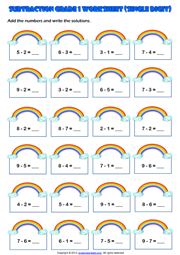Home Maths Main Page

# First Grade Single Digit Subtraction Math Exercise Worksheet

A simple single digit subtraction exercise maths worksheet for grade 1 (first grade) students and kids with rainbow theme. Subtract the numbers and write the solutions. (Horizontal Subtraction Worksheet for first grade students)Next Worksheet Grade 1 Subtraction Main Page Home